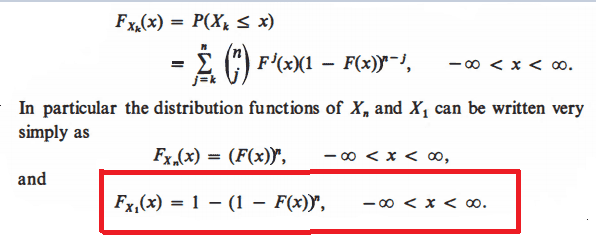# Distribution function

• MHB
• WMDhamnekar

#### WMDhamnekar

MHBNow, I don't understand how did author compute $F_{X_1}(x) = \displaystyle\sum_{j=1}^n \binom{n}{1} F^1(x) (1-F(x))^{n-1} = 1-(1-F(x))^n ?$ (I know L.H.S = R.H.S)

Would any member of Math help board explain me that? Any math help will be accepted.

The formula $\binom nj F^j(x)(1-F(x))^{n-j}$ is the probability mass function of the binomial distribution with parameters $p=F(x)$ and $n$.
Consequently we have that all possibilities for a specific $x$ sum up to $1$.
It implies that $F_{X_0}(x)=1$.
We can use the complement rule $P(A^c)=1-P(A)$ to calculate $F_{X_1}(x)$.